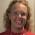Percent of change

When some quantity changes, such as a price or the amount of students, we can measure either the absolute change ("The price increased by \$5" or "There were 93 less students this year"), or the percent change.

In percent change, we express WHAT PART of the original quantity the change was.

For example, if a gadget costs \$44 and the price is increased by \$5, we measure the percent change by first considering WHAT PART \$5 is of \$44. Of course the answer is easy: it is 5/44 or five forty-fourths parts.

To make it percent change, however, we need to express that part using hundredths and not 44th parts. this happens to be easy, too. As seen in my previous post, you COULD make a proportion to find out how many hundredths 5/44 is:

5/44 = x/100

To solve this, you simply go 5/44 x 100, which is easy enough to remember in itself. In fact, this is the rule often given: you compare the PART to the WHOLE using division (5/44), and multiply that by 100.

There were 568 students one year, and 480 the next year. By how may percent did the student population decrease?

You first calculate the absolute change, which is 568 − 480 = 88. Then we find what part of the original population is 88 (it is 88/568), and express that using hundredth parts (percents):

88/568 x 100 = 15.49%

The student population decreased by 15.49%.

Often we are given the opposite problem: we know the percent of change and the original situation, and are asked about the new situation.

The price was \$4.55 and increased by 14.78%. What is the new price?

Here, we'd need to find the price increase, or the absolute change in price first. We know the percent part of the total (it is 14.78/100) and the total amount, so multiplying those we get the part as a dollar-amount: 14.78/100 x \$4.55 = \$0.67249. So this is the increase. To find the new price, add the increase to the original: \$4.55 + \$0.67249 = \$5.22249 = \$5.22.

Instead of multiplying by 14.78/100, it is far quicker to multiply by 0.1478 — or to change the percent-amount 14.78% to a decimal 0.1478 and multiply by it.

And, since in the end we need to add the original total, the whole calculation looks like this:

0.1478 × \$4.55 + \$4.55

Here, using distributive property we can make it look like this:

= \$4.55 (0.1478 + 1) = \$4.55(1.1478) = 1.1478 × \$4.55

So it can all be done in one multiplication. Instead of multiplying by the decimal 0.1478, you add 1 to it before multiplying.

Then one more possible problem type is that you know the percent of change and the actual change amount (absolute change), and are asked the original and/or the new total.

The price increased by 13%, or by \$10.14. What was the original price?

Let the original price be p. Then you can build an equation based on the idea that the price increase is 13% of p:

0.13p = \$10.14   or   13/100p = \$10.14

p = \$10.14/0.13 = \$78.

The new price would be found by adding.

Some other lessons to read are below:

Percent Of Change - Lesson and Problems

General Increase and Decrease Examples from Purplemath.com

Percent of change calculator - enter the original and the changed quantities, and it calculates the percent of change.

PERCENT INCREASE OR DECREASE lesson from TheMathPage.comAnonymous said…Anonymous said…
What if you know the percent of change and the new price, but you dont know the original price?Maria Miller said…
For example if some unknown price x increased by 20% and the new price is \$80:

1.2x = 80

x = 80/1.2

x = 66.67Anonymous said…
What is the reasoning behind adding the one to the percentage (ie: 1.2). I know what it does that it adds the percentage to the original number but I don't get whyAnonymous said…
To pm1101....

They have already done some of the algrba for you. Think of the problem this way. Price x is added to 20% of price x to yeild the new price of 80.

x+.2x=80

(1+.2)x=80

1.2x=80BLS said…
Thank you so much Maria. I was helping my younger brother to calculate the original amount after a percentage increase, and I had forgot it is 1.2x=80 and I kept doing .2x=80...Thank a Ton!!!!! This blog is great!Reneliaz said…
ThxAnonymous said…
I was having difficulties with these type of questions but im getting the hang of it now.
Great help, thank you maria!Fore Siu said…
im now upset about wt is the purpose of using percentage changes instead of actual changesAnonymous said…
This has really helped me do my math homework!!!Thanks sooo much! You explain it way better than my own math teacher!Anonymous said…
the discounted price is 24.01 and it was 30% off how do i find the orginal proceMaria Miller said…
If the discounted price is 24.01, and it is 30% off, then the discounted price is 70% of the original (70% of the price is left when 30% is taken off).

If 24.01 is 70% of it,.... then
24.01 ÷ 7 gives us 10% of the original price.

24.01 ÷ 7 = 3.43.

That times ten gives you 100% of the price. So the original price is 34.30.alex said…
thank u for all your help on percent changeAnonymous said…
I tried adding the one and it worked but only if the percent was increasing; it didn't work when the percent was decreasing. Help?Maria Miller said…
When it is decreasing you subtract from 1,instead of adding 1 to it.